Related Articles
Getting started with Machine Learning
• Difficulty Level : Easy
• Last Updated : 11 May, 2020

This article discusses the categories of machine learning problems, and terminologies used in the field of machine learning.

Types of machine learning problems

There are various ways to classify machine learning problems. Here, we discuss the most obvious ones.
1. On basis of the nature of the learning “signal” or “feedback” available to a learning system

• Supervised learning: The computer is presented with example inputs and their desired outputs, given by a “teacher”, and the goal is to learn a general rule that maps inputs to outputs. The training process continues until the model achieves the desired level of accuracy on the training data. Some real-life examples are:
• Image Classification: You train with images/labels. Then in the future you give a new image expecting that the computer will recognize the new object.
• Market Prediction/Regression: You train the computer with historical market data and ask the computer to predict the new price in the future.
• Unsupervised learning: No labels are given to the learning algorithm, leaving it on its own to find structure in its input. It is used for clustering population in different groups. Unsupervised learning can be a goal in itself (discovering hidden patterns in data).
• Clustering: You ask the computer to separate similar data into clusters, this is essential in research and science.
• High Dimension Visualization: Use the computer to help us visualize high dimension data.
• Generative Models: After a model captures the probability distribution of your input data, it will be able to generate more data. This can be very useful to make your classifier more robust.

A simple diagram which clears the concept of supervised and unsupervised learning is shown below: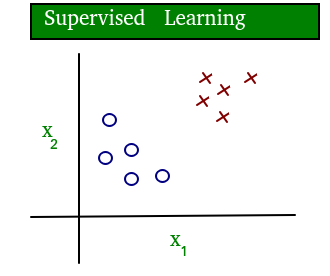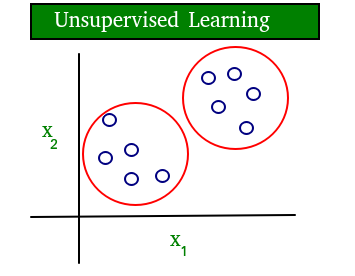As you can see clearly, the data in supervised learning is labelled, where as data in unsupervised learning is unlabelled.

• Semi-supervised learning: Problems where you have a large amount of input data and only some of the data is labeled, are called semi-supervised learning problems. These problems sit in between both supervised and unsupervised learning. For example, a photo archive where only some of the images are labeled, (e.g. dog, cat, person) and the majority are unlabeled.
• Reinforcement learning: A computer program interacts with a dynamic environment in which it must perform a certain goal (such as driving a vehicle or playing a game against an opponent). The program is provided feedback in terms of rewards and punishments as it navigates its problem space.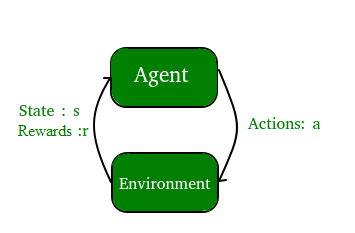2. On the basis of “output” desired from a machine learned system

• Classification: Inputs are divided into two or more classes, and the learner must produce a model that assigns unseen inputs to one or more (multi-label classification) of these classes. This is typically tackled in a supervised way. Spam filtering is an example of classification, where the inputs are email (or other) messages and the classes are “spam” and “not spam”.
• Regression: It is also a supervised learning problem, but the outputs are continuous rather than discrete. For example, predicting the stock prices using historical data.

An example of classification and regression on two different datasets is shown below: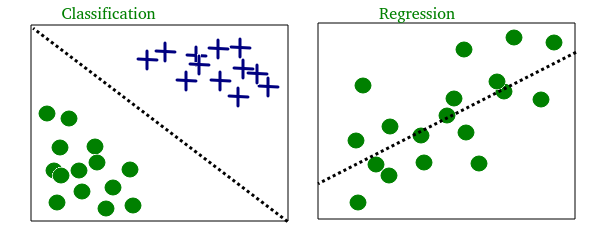• Clustering: Here, a set of inputs is to be divided into groups. Unlike in classification, the groups are not known beforehand, making this typically an unsupervised task.
As you can see in the example below, the given dataset points have been divided into groups identifiable by the colors red, green and blue.
•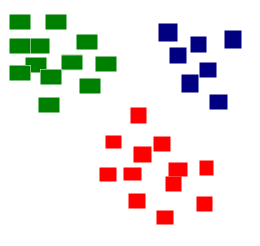• Density estimation: The task is to find the distribution of inputs in some space.
• Dimensionality reduction: It simplifies inputs by mapping them into a lower-dimensional space. Topic modeling is a related problem, where a program is given a list of human language documents and is tasked to find out which documents cover similar topics.

On the basis of these machine learning tasks/problems, we have a number of algorithms which are used to accomplish these tasks. Some commonly used machine learning algorithms are Linear Regression, Logistic Regression, Decision Tree, SVM(Support vector machines), Naive Bayes, KNN(K nearest neighbors), K-Means, Random Forest, etc.

Note: All these algorithms will be covered in upcoming articles.

Terminologies of Machine Learning

• Model
A model is a specific representation learned from data by applying some machine learning algorithm. A model is also called hypothesis.
• Feature
A feature is an individual measurable property of our data. A set of numeric features can be conveniently described by a feature vector. Feature vectors are fed as input to the model. For example, in order to predict a fruit, there may be features like color, smell, taste, etc.
Note: Choosing informative, discriminating and independent features is a crucial step for effective algorithms. We generally employ a feature extractor to extract the relevant features from the raw data.
• Target (Label)
A target variable or label is the value to be predicted by our model. For the fruit example discussed in the features section, the label with each set of input would be the name of the fruit like apple, orange, banana, etc.
• Training
The idea is to give a set of inputs(features) and it’s expected outputs(labels), so after training, we will have a model (hypothesis) that will then map new data to one of the categories trained on.
• Prediction
Once our model is ready, it can be fed a set of inputs to which it will provide a predicted output(label).

The figure shown below clears the above concepts: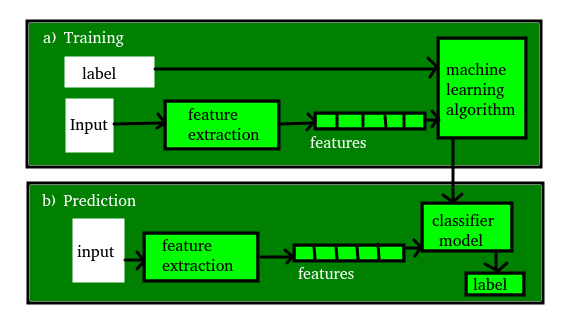Related Articles:

References:

This blog is contributed by Nikhil Kumar. If you like GeeksforGeeks and would like to contribute, you can also write an article using contribute.geeksforgeeks.org or mail your article to contribute@geeksforgeeks.org. See your article appearing on the GeeksforGeeks main page and help other Geeks.